## Resource of the Week: know number facts in year 2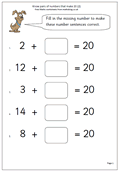A lot is expected of children in Year 2, especially what they should know off by heart when calculating. By the end of the year they should:

•    know all addition facts for two numbers up to a total of 10.      (eg 4 + 5.)

•    be able to derive subtraction facts for numbers up to 10.     (eg if they know that 6 + 3 = 9, they can instantly work out that 9 – 6 = 3.)

•    know all the pairs of whole numbers which total 20.    (eg 16 + 4.)

•    know all the pairs of multiples of 10 which total 100.   (eg 30 + 70.)

•    know the doubles of whole numbers up to a total of 20.  (eg double 7.)

•    understand that halving is the inverse of doubling and hence derive halving facts from their knowledge of   doubling.   (eg if double 8 is 16, then half 16 is 8.)

•    recall 2, 5 and 10 times-tables.  (eg know 5 x 6.)

•    work out related division facts.   (eg if 5 times 2 is 10, then 10 divided by 5 is 2.)

Now, that is quite a lot to know with instant recall, and they will need plenty of practice to achieve this. Why not go to our Year 2 Knowing and Using Number facts to help them on their way?

Year 2 Knowing Number Facts

## Resource of the Week: Writing larger numbers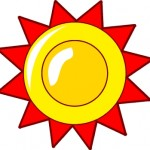Writing large numbers in words is not easy but by the end of year 5 many children will be confident enough to read numbers in the millions.

In the past, in the UK, a billion has been considered as a million million, but in the USA a thousand million has been thought of as a billion.

This seems to be the norm in the UK these days as well so Neptune at 4 500 000 000 km from the sun can be said as “four billion, five hundred million km “.

Some children become fascinated by larger numbers and like to count on up to trillions and beyond, even to the googol which is a 1 with one hundred zeros!

(10,­000,­000,­000,­000,­000,­000,­000,­000,­000,­000,­000,­000,­000,­000,­000,­000,­000,­000,­000,­000,­000,­000,­000,­000,­000,­000,­000,­000,­000,­000,­000,­000,­000)

Free Y5 maths worksheet: writing larger numbers

## Year 5 Maths Worksheet: Square numbers (4)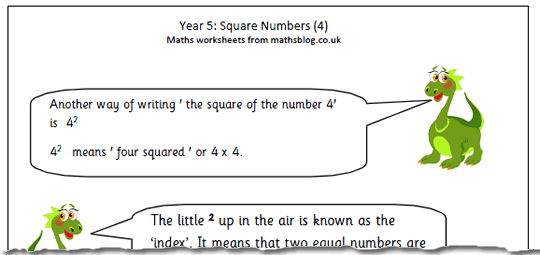This maths worksheet for Year 5 children introduces the idea of  ‘index’ for writing the square of a number. Children should understand that 42 means ‘4 squared’ or 4 times 4. Often it is mistaken for 4 x 2.

Remind children that ‘square numbers’ are called ‘square numbers because they can be represented in an array with an equal number in both rows and columns. Again, encourage children to learn their square numbers up to at least 10 squared. This will help greatly when square roots are introduced.

Square numbers (4)

## Year 5 Maths Worksheets: Square numbers (3)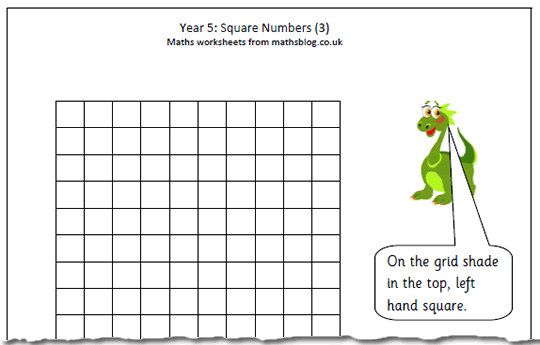‘Maths is all about patterns!’ said my maths lecturer over 30 years ago and this worksheet on square numbers proves just how right he was. It uses a blank 10×10 square to show the pattern made when producing square numbers.

It is interesting to note that the second square number (4) is made by adding the first two odd numbers (1 + 3) and the third square number is made up of the first three odd numbers (1 + 3 + 5 = 9). This pattern continues (for ever!) with the fourth square number (16) made up of (1 + 3 + 5 + 7) and so on. By colouring in the number square it should be apparant to children why this is happening.

Square numbers 3

## Year 5 Maths Worksheet: Square Numbers (1)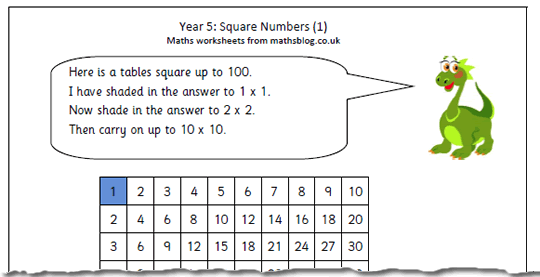This maths worksheet uses a 10×10 tables square to show the patterns involved with square numbers. All the square numbers lie diagonally from top left to bottom right.

Once children have seen the pattern and worked out all the square numbers from 1 to 100 it is a good idea to encourage them to learn the numbers off by heart, as if in a list.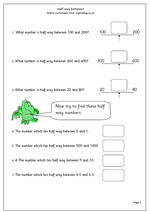Finding the number which lies half way between two other numbers can be easy or quite tricky, even when using fairly small numbers. When using whole tens or hundreds the questions tend to be quite straightforward, as does finding halfway between two consecutive whole numbers. Most of the questions on this number worksheet are of this easier type. One of the harder questions here is to find half way between 20 and 80 and it is interesting to ask how people go about this mentally. Often they will conduct a mental process of adding ten to the lower number and subtracting ten from the higher number until they meet ‘in the middle’.